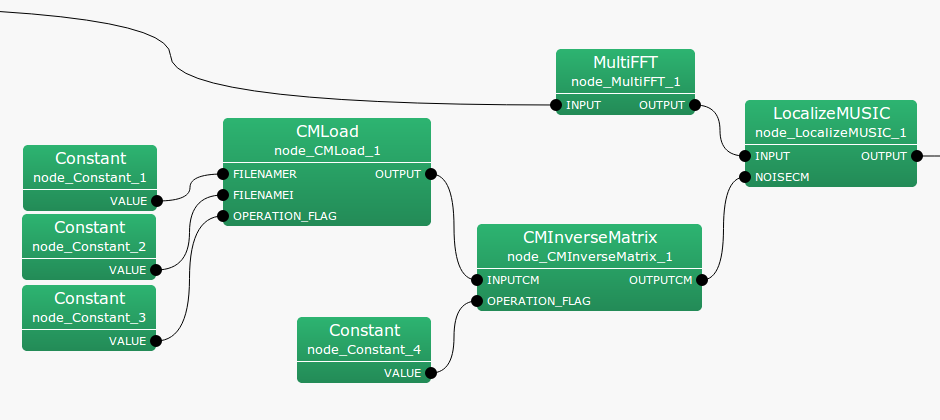## 6.2.9 CMInverseMatrix

### 6.2.9.1 Module Overview

Calculates the inverse of the sound source correlation matrix.

None.

### 6.2.9.3 Usage

In what case is the node used?

The calculation node of the correlation matrix is the one created for the sound source from CMMakerFromFFT , CMMakerFromFFTwithFlag , and has the function to calculate the inverse matrix of the correlation matrix.

Typical Examples

Figure. 6.25 shows a usage example of CMInverseMatrix  node.

INPUTCM input terminal is connected to a correlation matrix calculated from CMMakerFromFFT  or CMMakerFromFFTwithFlag , etc. (type is Matrix<complex<float> >  type, but to handle a correlation matrix, convert the three dimensional complex array to a two dimensional complex array and then output). OPERATION_FLAG is int  type or bool  type input, and specifies when to calculate the inverse matrix of the correlation matrix.Figure 6.25: Network Example using CMInverseMatrix

### 6.2.9.4 I/O and property setting of the node

Table 6.24: Parameter list of CMInverseMatrix
 Parameter Type Default Unit Description FIRST_FRAME_EXECUTION false Selection of the first frame execution ENABLE_DEBUG false ON/OFF of debugging information output

Input

INPUTCM

: Matrix<complex<float> >  type. A correlation matrix of each frequency bin. The $M$-th order complex square array correlation matrix outputs $NFFT/2 + 1$ items. Matrix<complex<float> >  contains rows corresponding to frequencies ($NFFT/2 + 1$ rows), and columns containing the complex correlation matrix ($M * M$ columns across).

OPERATION_FLAG

: int  type or bool  type. Only when this input terminal is 1 or when true, the calculation of correlation matrix is performed.

Output

OUTPUTCM

: Matrix<complex<float> >  type. The correlation matrix after the inverse matrix calculation is output.

Parameter

FIRST_FRAME_EXECUTION

: bool  type. Default value is false. When true, OPERATION FLAG is always 0. Even when it is false, the operation is performed only on the first frame.

ENABLE_DEBUG

: bool  type. Default value is false. When true, output the frame number calculated to the standard output, during correlation matrix calculation.

### 6.2.9.5 Module Description

Calculates the inverse matrix of the correlation matrix. The correlation matrix is a complex three-dimensional array of size $k \times M \times M$ and the inverse matrix calculation $k$ times is performed as follows. Here, $k$ is the number of frequency bins ($k = NFFT/2 + 1$), and $M$ is the number of channels in the input signal.


OUTPUTCM = zero_matrix(k,M,M)

calculate{

IF OPERATION_FLAG
FOR i = 1 to k
OUTPUTCM[i] = inverse( INPUTCM[i] )
ENDFOR
ENDIF

}


The matrix that is output from OUTPUTCM terminal is initialized as a zero matrix, and maintains the final operational result from this point onward.